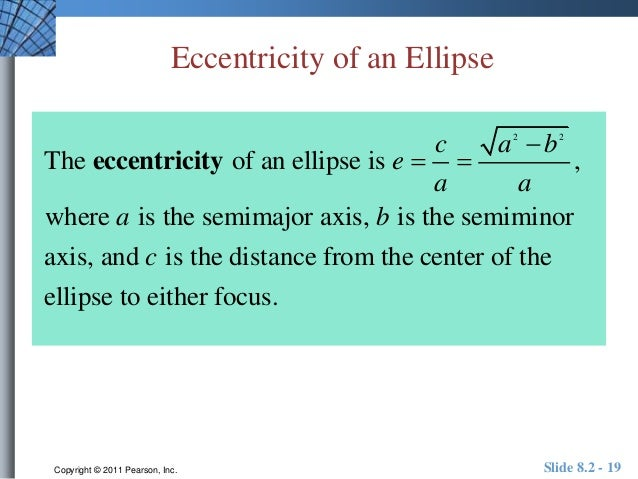User Name Remember Me? Password

 Advanced Mechanics Advanced Mechanics Physics Help ForumAug 6th 2017, 06:26 PM #1 Senior Member   Join Date: Nov 2013 Location: New Zealand Posts: 550 Explorer 1 Orbit Exercise taken from Caiken, pg 23For part (a) I get (350+2549+2*6378)/2 = 7287.5 km for part (b) ... Used formula for conic sections $\displaystyle \frac{p}{r} = 1 + e \cos \theta$ assumed perigee when $\displaystyle \cos \theta = 0$ and apogee when $\displaystyle cos \theta = 1$. This gives two linear equations: p/(360+6378) - e = 1 p/(2549+6378) + e = 1 I get p = 7679.55646345356 and e = 0.139738270028726 for part (c) I get a bit stuck. I can't think of any formula that will help without knowing the mass of the satellite. Edit: Looks like Keplers 3rd law is a possible candidate for (c) $\displaystyle T^2 = \frac{4 \pi^2}{G M}a^3$ where a is the semi major axis and T is the period. That's a step closer anyway. Last edited by kiwiheretic; Aug 7th 2017 at 01:18 AM.Aug 7th 2017, 07:27 AM #2 Senior Member   Join Date: Aug 2008 Posts: 113 part (a) ... if the Earth's equatorial radius is 6378 km, the the semi-major axis is a = [360+2549+2(6378)]/2 = 7832.5 km part (b) ... focal length, c = 7832.5 - (360+6378) = 1094.5 km e = c/a = 1094.5/7832.5 = 0.1397... part (c) ... at the Earth's surface, $g = 9.81 \, m/s^2$ $g = \dfrac{GM}{R_e^2} \implies GM = g \cdot R_e^2$ $T^2 = \dfrac{4\pi^2}{GM} \cdot a^3 = \dfrac{4\pi^2}{g \cdot R_e^2} \cdot a^3$Aug 7th 2017, 07:18 PM #3 Senior Member   Join Date: Nov 2013 Location: New Zealand Posts: 550 Looks like you did something different for eccentricity that didn't involve a system of linear equations? you got a different answer for (a) but used same numbers? I just double checked with IPython as a calculator. Last edited by kiwiheretic; Aug 7th 2017 at 07:20 PM.Aug 8th 2017, 05:03 PM   #4
Senior Member

Join Date: Aug 2008
Posts: 113
 Originally Posted by kiwihereticLooks like you did something different for eccentricity that didn't involve a system of linear equations?you got a different answer for (a) but used same numbers? I just double checked with IPython as a calculator.
get a new calculator ...Aug 8th 2017, 05:22 PM   #5
Senior Member

Join Date: Nov 2013
Location: New Zealand
Posts: 550
 Originally Posted by kiwihereticFor part (a) I get (350+2549+2*6378)/2 = 7287.5 km
Oh, was a typo, I used a 350 instead of 360Aug 8th 2017, 05:56 PM   #6
Senior Member

Join Date: Aug 2008
Posts: 113
 Originally Posted by kiwihereticOh, was a typo, I used a 350 instead of 360
 For part (a) I get (350+2549+2*6378)/2 = 7287.5 km
you also transposed the digits 2 and 8Tags explorer, orbitThread ToolsShow Printable VersionEmail this Page Display ModesLinear ModeSwitch to Hybrid ModeSwitch to Threaded ModeSimilar Physics Forum Discussions Thread Thread Starter Forum Replies Last Post kiwiheretic Nuclear and Particle Physics 15 Jul 1st 2014 03:30 PM nevermissedabeat Periodic and Circular Motion 5 Mar 21st 2014 11:11 PM afwings Physics 0 Aug 26th 2010 10:05 AM C.E Advanced Mechanics 3 May 6th 2009 11:45 PM vasilicus Kinematics and Dynamics 0 Jan 18th 2009 05:17 PM